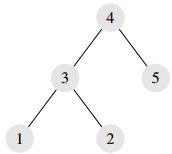## Day 1, Problem 3: LHC

For your latest top-secret experiment, you will need a large quantity of Higgs bosons. To obtain these elusive particles, you will need to build a large hadron collider, a long circular tunnel that you can use to accelerate particles and smash them into each other.

You already have access to an extensive network of tunnels, which is guaranteed to be connected and free of cyclic paths. In other words, the existing tunnels form a tree structure. This system can be represented by N junctions, labelled 1 through N, connected by N - 1 tunnels, each of which connects two junctions. Tunnels can be traversed in either direction (i.e., if there is a junction from a to b, that junction also goes from b to a).

By adding exactly one tunnel to the network, you can create a cyclic path, which you will use to build your large hadron collider. You wish to form the longest possible collider in this way, where we define length as the number of tunnels in a cycle. Also, you would like to compute the number of ways to do this– that is, the number of distinct pairs of junctions such that adding a tunnel between them forms a cycle of maximum length.

For example, in the following network, we can form a collider of length 4 by building a tunnel between junctions 1 and 5, or between 2 and 5:### Input

The first line of each test case will contain N (3 ≤ N ≤ 400 000), the number of junctions. The next N - 1 lines will each contain two space-separated integers i and j, indicating that there is a tunnel between junctions i and j.

### Output

The output should consist of a single line with two space-separated integers: the length of the longest possible collider, and the number of ways to achieve that length. Note that you may need a 64-bit integer to store the answer (`long long` in C/C++, `LongInt` in Pascal).

For test cases worth 40% of the points, you may assume N ≤ 5000.

```5
1 3
2 3
3 4
4 5```

### Sample Output

`4 2`

Point Value: 17 (partial)
Time Limit: 5.00s
Memory Limit: 64M
Author: Cyril

Languages Allowed:
C++03, PAS, C, HASK, ASM, RUBY, PYTH2, JAVA, PHP, SCM, CAML, PERL, C#, C++11, PYTH3

``int64``
``longint``